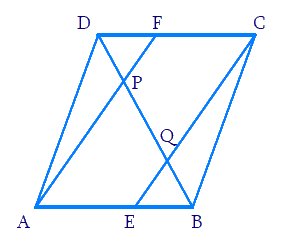# Ex.8.2 Q5 Quadrilaterals Solution - NCERT Maths Class 9

Go back to  'Ex.8.2'

## Question

In a parallelogram $$ABCD$$, $$E$$ and $$F$$ are the mid-points of sides $$AB$$ and $$CD$$ respectively (see the given figure). Show that the line segments $$AF$$ and $$EC$$ trisect the diagonal $$BD$$.Video Solution
Ex 8.2 | Question 5

## Text Solution

What is known?

In a parallelogram $$ABCD$$, $$E$$ and $$F$$ are the mid-points of sides $$AB$$ and $$CD$$ respectively.

What is unknown?

How we can show that the line segments $$AF$$ and $$EC$$ trisect the diagonal $$BD.$$

Reasoning:

In a quadrilateral if one pair of opposite sides is parallel and equal to each other. Then it is a parallelogram. Also by converse of mid-point theorem, we know that a line drawn through the mid-point of any side of a triangle and parallel to another side, bisects the third side.

Steps:

$$ABCD$$ is a parallelogram.

$$AB$$ $$\parallel$$ $$CD$$

And hence, $$AE$$ $$\parallel$$ $$FC$$

Again, $$AB = CD$$ (Opposite sides of parallelogram $$ABCD$$)

\begin{align}\frac{1}{2}{AB}=\frac{1}{2}{CD}\end{align}

$$AE = FC$$ ($$E$$ and $$F$$ are mid-points of side $$AB$$ and $$CD$$)

In quadrilateral $$AECF$$, one pair of opposite sides ($$AE$$ and $$CF$$) is parallel and equal to each other. Therefore, $$AECF$$ is a parallelogram.

$$\therefore$$  $$AF$$ $$\parallel$$ $$EC$$ (Opposite sides of a parallelogram)

In $$\rm \Delta DQC,$$ $$F$$ is the mid-point of side $$DC$$ and $$FP$$ $$\parallel$$ $$CQ$$ (as $$AF$$ $$\parallel$$ $$EC$$). Therefore, by using the converse of mid-point theorem, it can be said that $$P$$ is the mid-point of $$DQ$$.

$$\therefore$$ $$DP = PQ$$... (1)

Similarly, in $$\rm \Delta APB,$$ $$E$$ is the mid-point of side $$AB$$ and $$EQ$$ $$\parallel$$ $$AP$$ (as $$AF$$ $$\parallel$$ $$EC$$).

Therefore, by using the converse of mid-point theorem, it can be said that $$Q$$ is the mid-point of $$PB$$.

$$\therefore$$ $$PQ = QB$$ ... (2)

From Equations (1) and (2),

$$DP = PQ = BQ$$

Hence, the line segments $$AF$$ and $$EC$$ trisect the diagonal $$BD$$.

Video Solution